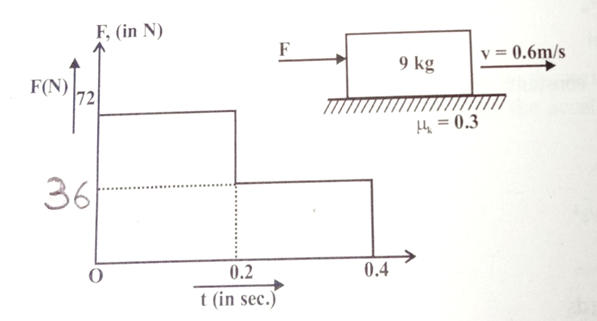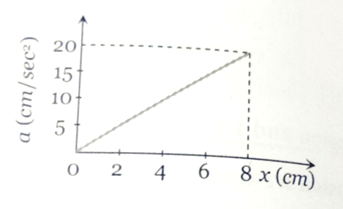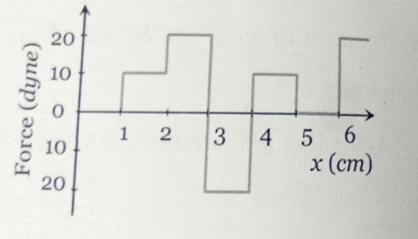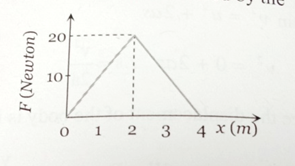The acceleration of a particle is given by a=3t and at t=0, v=0, x=0. The velocity and displacement at t = 2 sec will be-

1. 6 m/s, 4 m

2. 4 m/s, 6 m

3. 3 m/s, 2 m

4. 2 m/s, 3 m

Concept Questions :-

Integration
High Yielding Test Series + Question Bank - NEET 2020

Difficulty Level:

The 9 kg block is moving to the right with a velocity of 0.6 m/s on a horizontal surface when a force F, whose time variation is shown in the graph, is applied to it at time t = 0. Calculate the velocity v of the block when t= 0.4s. The coefficient of kinetic fricton is ${\mu }_{k}=0.3$[ This question is only for Dropper and XII batch]1. 0.6 m/s

2. 1.2 m/s

3. 1.8 m/s

4. 2.4 m/s

Concept Questions :-

Integration
High Yielding Test Series + Question Bank - NEET 2020

Difficulty Level:

A 10 kg mass moves along x-axis. Its acceleration as a function of its position is shown in the figure. What is the total work done on the mass by the force as the mass moves from x=0 to x=8 cm [This question is only for Dropper and XII batch]1.

2.

3.

4.

Concept Questions :-

Integration
High Yielding Test Series + Question Bank - NEET 2020

Difficulty Level:

The relationship between force and position is shown in the figure given (in one dimensional case). Find the work done by the force in displaying a body from x= 1 cm to x= 5cm is   [This question is only for Dropper and XII batch]1. 10 erg

2. 20 erg

3. 30 erg

4. 40 erg

Concept Questions :-

Integration
High Yielding Test Series + Question Bank - NEET 2020

Difficulty Level:

The graph between the resistive force F acting on a body and the distance covered by the body is shown in the figure. The mass of the body is 25 kg and initial velocity is 2 m/s. When the distance covered by the body is 4 m, its kinetic energy would be    [This question is only for Dropper and XII batch]1. 50 J

2. 40 J

3. 20 J

4. 10 J

Concept Questions :-

Integration
High Yielding Test Series + Question Bank - NEET 2020

Difficulty Level:

A long spring is stretched by 2 cm, its potential energy is U. If the spring is streched by 10 cm, find the potential energy stored in it.   [This question is only for Dropper and XII batch]

1. 10 U

2. 15 U

3. 20 U

4. 25 U

Concept Questions :-

Integration
High Yielding Test Series + Question Bank - NEET 2020

Difficulty Level:

A spring of spring constant  is stretched initially by 5 cm from the unstretched position. Find the work required to stretch it further by another 5 cm is   [This question is only for Dropper and XII batch]

1. 15 J

2. 18.75 N .m

3. 20 J

4. 22.75 N .m

Concept Questions :-

Integration
High Yielding Test Series + Question Bank - NEET 2020

Difficulty Level:

A constant force F is applied on a body. The power (P) generated is related to the time elapsed (t) as

[This question is only for Dropper and XII batch]

1.

2.

3.

4.

Concept Questions :-

Integration
High Yielding Test Series + Question Bank - NEET 2020

Difficulty Level:

The gravitational field due to a mass distribution is given by , where k is a constant. Assuming the potential to be zero at infinity, find the potential at a point x = a. [This question is only for Dropper and XII batch]

1. $\frac{k}{{a}^{2}}$

2. $\frac{-k}{{a}^{2}}$

3. $\frac{k}{a}$

4. $\frac{-k}{a}$

Concept Questions :-

Integration
High Yielding Test Series + Question Bank - NEET 2020

Difficulty Level:

Electric field is given by E $=\frac{100}{{x}^{2}}$ . Find the potential difference between x= 10 and x= 20 m. [This question is only for Dropper and XII batch]

1. 5 V

2. 10 V

3. 15 V

4. 20 V

Concept Questions :-

Integration